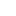# Total expenditure, average expenditure and distribution of household expenditure

1.1 Total expenditure, average expenditure and percentage distribution of expenditure according to different levels of functional breakdown
1.Última modificación:
06/29/2021
Dimensiones:
Periodo:
1.1 Total expenditure and average expenditure by expenditure group (2 digits).
2.Última modificación:
06/29/2021
Dimensiones:
Periodo:
1.2 Total expenditure and average expenditure by expenditure subgroup (3 digits ECOICOP/EPF).
3.Última modificación:
06/29/2021
Dimensiones:
Periodo:
1.3 Total expenditure and average expenditure by expenditure code (4 digits ECOICOP/EPF).
4.Última modificación:
06/29/2021
Dimensiones:
Periodo:
1.4 Total expenditure and average expenditure by expenditure code (5 digits ECOICOP/EPF).
5.Última modificación:
06/29/2021
Dimensiones:
Periodo:
1.5 Total expenditure and average expenditure by expenditure subgroup (3 digits ECOICOP).
6.Última modificación:
06/29/2021
Dimensiones:
Periodo:
1.6 Total expenditure and average expenditure by expenditure code (4 digits ECOICOP).
7.Última modificación:
06/29/2021
Dimensiones:
Periodo:
1.7 Total expenditure and average expenditure by expenditure code (5 digits ECOICOP).
2.2 Total expenditure, average expenditure and percentage distribution of total expenditure and index on the mean of the average expenditure per person (a.e.p) according to different classification variables
3.3 Average expenditure per household, average expenditure per person and percentage distribution of total expenditure (2 digits) according to different classification variables
4.4 Percentage distribution of expenditure by expenditure subgroup (3 digits), according to different classification variables
5.5 Average expenditure per household, average expenditure per person and percentage of households with expenditure in certain codes (list of codes up to 5 digits ECOICOP/EPF)
6.6 Means household expense, mean expense per person and distribution of the expense (vertical and horizontal percentages) by expense groups and by quintile of the expense
7.7 Expenditure on Internet purchases